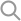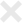PredictionIO enables developer to implement evaluation custom evaluation metric with just a few lines of code. We illustrate it with the classification template.

## Overview

A simplistic form of metric is a function which takes a (Query, PredictedResult, ActualResult)-tuple (QPA-tuple) as input and return a score. Exploiting this properties allows us to implement custom metric with a single line of code (plus some boilerplates). We demonstrate this with two metrics: accuracy and precision.

## Example 1: Accuracy Metric

Accuracy is a metric capturing the portion of correct prediction among all test data points. A way to model this is for each correct QPA-tuple, we give a score of 1.0 and otherwise 0.0, then we take an average of all tuple scores.

PredictionIO has a [[AverageMetric]] helper class which provides this feature. This class takes 4 type parameters, [[EvalInfo]], [[Query]], [[PredictedResult]], and [[ActualResult]], these types can be found from the engine's signature. Line 5 below is the custom calculation.

 1 2 3 4 5 6 case class Accuracy extends AverageMetric[EmptyEvaluationInfo, Query, PredictedResult, ActualResult] { def calculate(query: Query, predicted: PredictedResult, actual: ActualResult) : Double = (if (predicted.label == actual.label) 1.0 else 0.0) } 

Once we define a metric, we tell PredictionIO we are using it in the Evaluation object. We can run the following command to kick start the evaluation.

 1 2 3 4 $pio build ...$ pio eval org.example.classification.AccuracyEvaluation org.example.classification.EngineParamsList ... 

(See MyClassification/src/main/scala/Evaluation.scala for full usage.)

## Example 2: Precision Metric

Precision is a metric for binary classifier capturing the portion of correction prediction among all positive predictions. We don't care about the cases where the QPA-tuple gives a negative prediction. (Recall that a binary classifier only provide two output values: positive and negative.) The following table illustrates all four cases:

PredictedResult ActualResult Value
Positive Positive 1.0
Positive Negative 0.0
Negative Positive Don't care
Negative Negative Don't care

Calculating the precision metric is a slightly more involved procedure than calculating the accuracy metric as we have to specially handle the don't care negative cases.

PredictionIO provides a helper class OptionAverageMetric allows user to specify don't care values as None. It only aggregates the non-None values. Lines 3 to 4 is the method signature of calculate method. The key difference is that the return value is a Option[Double], in contrast to Double for AverageMetric. This class only computes the average of Some(.) results. Lines 5 to 13 are the actual logic. The first if factors out the positively predicted case, and the computation is similar to the accuracy metric. The negatively predicted case are the don't cares, which we return None.

 1 2 3 4 5 6 7 8 9 10 11 12 13 14 15 case class Precision(label: Double) extends OptionAverageMetric[EmptyEvaluationInfo, Query, PredictedResult, ActualResult] { def calculate(query: Query, predicted: PredictedResult, actual: ActualResult) : Option[Double] = { if (predicted.label == label) { if (predicted.label == actual.label) { Some(1.0) // True positive } else { Some(0.0) // False positive } } else { None // Unrelated case for calcuating precision } } } 

We define a new Evaluation object to tell PredictionIO how to use this new precision metric.

 1 2 3 object PrecisionEvaluation extends Evaluation { engineMetric = (ClassificationEngine(), new Precision(label = 1.0)) } 

We can kickstarts the evaluation with the following command, notice that we are reusing the same engine params list as before. This address the separation of concern when we conduct hyperparameter tuning.

 1 2 3 4 5 6 7 8 9 10 11 12 13 14 15 16 17 18 19 20 21 22 23 24 25 26 27 28 29 30 31 32 33 34 35 36 37 38 39 40 41 42 43 44 45 $pio build ...$ pio eval org.example.classification.PrecisionEvaluation org.example.classification.EngineParamsList ... [INFO] [CoreWorkflow$] Starting evaluation instance ID: SMhzYbJ9QgKkD0fQzTA7MA ... [INFO] [MetricEvaluator] Iteration 0 [INFO] [MetricEvaluator] EngineParams: {"dataSourceParams":{"":{"appId":19,"evalK":5}},"preparatorParams":{"":{}},"algorithmParamsList":[{"naive":{"lambda":10.0}}],"servingParams":{"":{}}} [INFO] [MetricEvaluator] Result: MetricScores(0.8846153846153846,List()) [INFO] [MetricEvaluator] Iteration 1 [INFO] [MetricEvaluator] EngineParams: {"dataSourceParams":{"":{"appId":19,"evalK":5}},"preparatorParams":{"":{}},"algorithmParamsList":[{"naive":{"lambda":100.0}}],"servingParams":{"":{}}} [INFO] [MetricEvaluator] Result: MetricScores(0.7936507936507936,List()) [INFO] [MetricEvaluator] Iteration 2 [INFO] [MetricEvaluator] EngineParams: {"dataSourceParams":{"":{"appId":19,"evalK":5}},"preparatorParams":{"":{}},"algorithmParamsList":[{"naive":{"lambda":1000.0}}],"servingParams":{"":{}}} [INFO] [MetricEvaluator] Result: MetricScores(0.37593984962406013,List()) [INFO] [CoreWorkflow$] Updating evaluation instance with result: MetricEvaluatorResult: # engine params evaluated: 3 Optimal Engine Params: { "dataSourceParams":{ "":{ "appId":19, "evalK":5 } }, "preparatorParams":{ "":{ } }, "algorithmParamsList":[ { "naive":{ "lambda":10.0 } } ], "servingParams":{ "":{ } } } Metrics: org.example.classification.Precision: 0.8846153846153846 

(See MyClassification/src/main/scala/PrecisionEvaluation.scala for the full usage.)